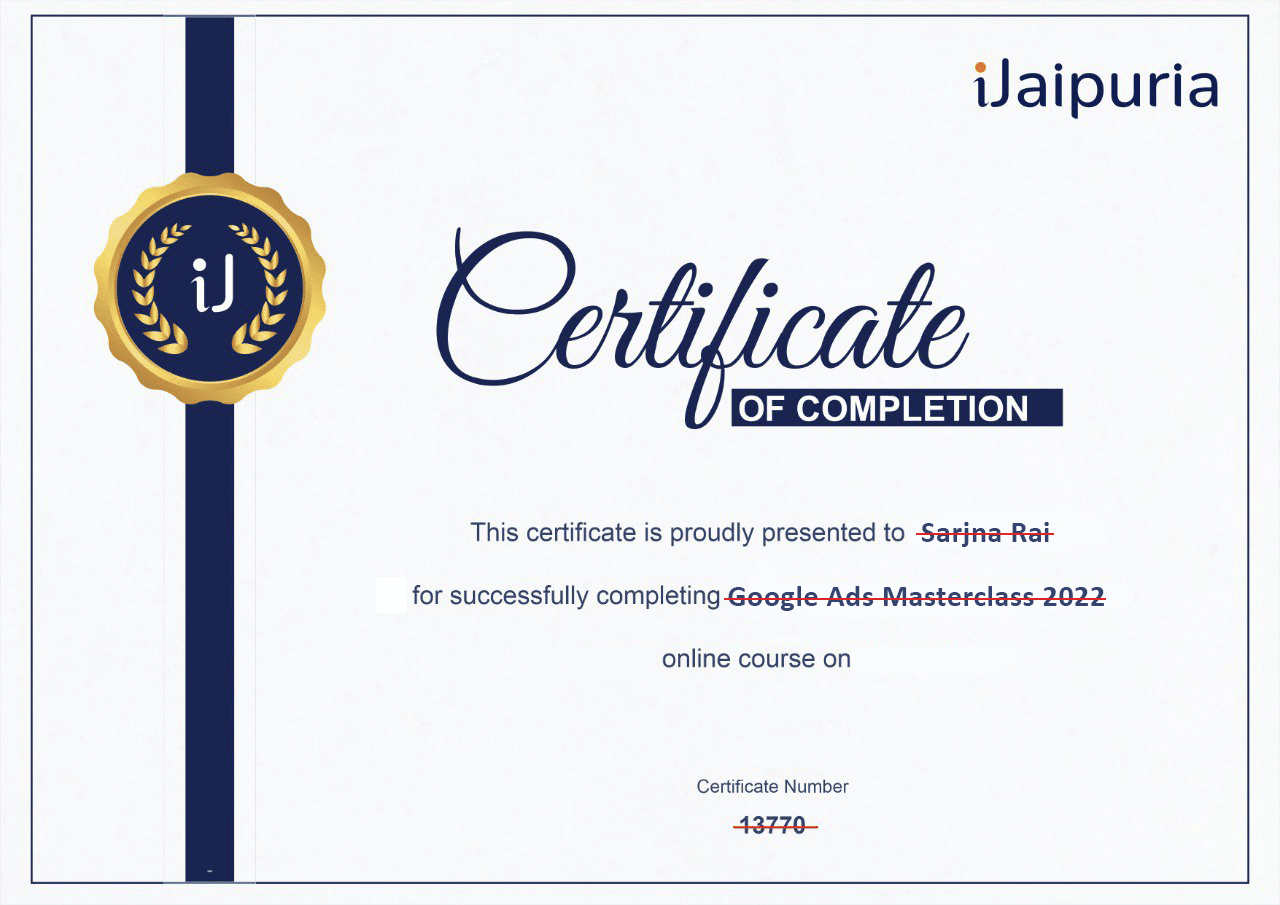###### Assured Certification
Rating (10634)
5/5## Course Structure

For every Artificial Intelligence, Machine Learning, and Data Science enthusiast, Statistics is fundamental to learning to dive deeper into these fields.

Correctly understanding Statistics will make it easy to implement regression, classification, and numerous other algorithms. This course is the combination of 4-5 available courses in the market, which will help you give you deeper insights and introduce you to various facets of statistics and concepts required to solve multiple data science problems.

With simplified and complete statistics training, quizzes, and practical steps – this is one of the most comprehensive Statistics courses available.

We’ll cover the fundamentals of statistics, Descriptive statistics, Inferential statistics, Hypothesis Testing, Correlation Analysis, Regression Analysis, Modelling, Ch- Squared tests, ANOVA, T-Test, Z Test, Index Number, Correlation, Regression and many more. By the end of this course, you will confidently implement techniques across the significant situations in Statistics, Business, and Data Analysis for research projects.

### Assured Certification

For every Artificial Intelligence, Machine Learning, and Data Science enthusiast, Statistics is fundamental to learning to dive deeper into these fields.

Correctly understanding Statistics will make it easy to implement regression, classification, and numerous other algorithms. This course is the combination of 4-5 available courses in the market, which will help you give you deeper insights and introduce you to various facets of statistics and concepts required to solve multiple data science problems.

With simplified and complete statistics training, quizzes, and practical steps – this is one of the most comprehensive Statistics courses available.

We’ll cover the fundamentals of statistics, Descriptive statistics, Inferential statistics, Hypothesis Testing, Correlation Analysis, Regression Analysis, Modelling, Ch- Squared tests, ANOVA, T-Test, Z Test, Index Number, Correlation, Regression and many more. By the end of this course, you will confidently implement techniques across the significant situations in Statistics, Business, and Data Analysis for research projects.

## Course FAQs

Statistics is one of the critical FIVE pillars of Data Science & AI. Knowledge of statistics not only helps in model (Algorithms) understanding but also lets you improve the performance of the models through parameters fine-tuning.

Statistics is a vast subject, and knowing everything about it demands massive effort and dedication. Luckily all the concepts of statistics are not required when it comes to Data Science & AI. However, you need to know basic statistical concepts like hypothesis testing and some crucial tests along with the concepts of central tendencies and probability density functions.

Some of the essential terms (but not limited to) include mean, median, mode, standard deviation, variance, skewness, kurtosis, central limit theorem, various statistical tests, descriptive statistics analysis, etc.

Hypothesis testing is a form of statistical inference that uses data from a sample to conclude a population parameter or a population probability distribution. First, a tentative assumption is made about the parameter or distribution. This assumption is called the null hypothesis and is denoted by H0.

Practising the relevant questions and implementing the concepts taught in the course in real case studies and real datasets will help you improve your overall confidence in this field.

## Course Structure

• Why Statistics Is Important For Data Science 05:58
• Important Terms In Statistics 12:06
• Types of Data & Hands-On Tutorial 18:40
• Central Tendency 21:19
• Mean
• Median
• Mode
• Variance 19:03
• Standard Deviation
• Expected value
• Distributions
• Histogram
• Analysis Plots 14:15
• Scatter Plot Analysis
• Box Plot Analysis
• Statistical Functions
• CLT-Central Limit Theorem
• Statistical Testing 17:52
• z test
• t Test
• R square Value 23:39
• ANOVA
• Regression Analysis 13:27
• Probability Functions 16:40
• Probability Mass Function
• Probability Density Function
• Correlation
• Statistical Testings 18:30
• Hypothesis Testing
• P Value
• Important Evaluation Metrics and Summary 13:28

## 1

### Concept

05:25 min
◉ Introduction To Statistics

## 2

### Mindset

05:58 min
◉ Why Statistics Is Important For Data Science

## 3

### Action

12:06 min
◉ Important Terms In Statistics

## 4

### Basics Of Statistics For Data Science & AI-Part 1

18:40 min
◉ Types of Data & Hands-On Tutorial

## 5

### Basics Of Statistics For Data Science & AI-Part 2

21:19 min
◉ Central Tendency
◉ Mean
◉ Median
◉ Mode

## 6

### Basics Of Statistics For Data Science & AI-Part 3

19:03 min
◉ Variance
◉ Standard Deviation
◉ Expected value
◉ Distributions
◉ Histogram

## 7

### Beyond The Basics Of Statistics For Data Science & AI-Part 1

14:15 min
◉ Analysis Plots
◉ Scatter Plot Analysis
◉ Box Plot Analysis
◉ Statistical Functions
◉ CLT-Central Limit Theorem

## 8

### Beyond The Basics Of Statistics For Data Science & AI-Part 2

17:52 min
◉ Statistical Testing
◉ z test
◉ t test

23:39 min
◉ R square Value
◉ ANOVA

## 10

### Beyond The Basics Of Statistics For Data Science & AI-Part 4

13:27 min
◉ Regression Analysis

## 11

### Beyond The Basics Of Statistics For Data Science & AI-Part 5

16:40 min
◉ Probability Functions
◉ Probability Mass Function
◉ Probability Density Function
◉ Correlation

## 12

### Beyond The Basics Of Statistics For Data Science & AI-Part 6

18:30 min
◉ Statistical Testing
◉ Hypothesis Testing
◉ P Value

## 13

### Important Evaluation Metrics and Summary

13:28 min
◉ Summary & ConclusionSachichidanand Kumar is a data scientist and AI practitioner. He is also a career coach with over 10 years of industrial experience in top MNCs and a PhD in computer science. Alumni of NIT and XLRI, he is also the co-founder of Fetchr-School Of Excellence Academy.

##### Sachichidanand Kumar

Data Science & AI Expert

## After finishing the course, you will get a Certificate of Completion.

#### Demonstrate Your CommitmentBe a growth-driven professional and advance yourcareer by learning new skills